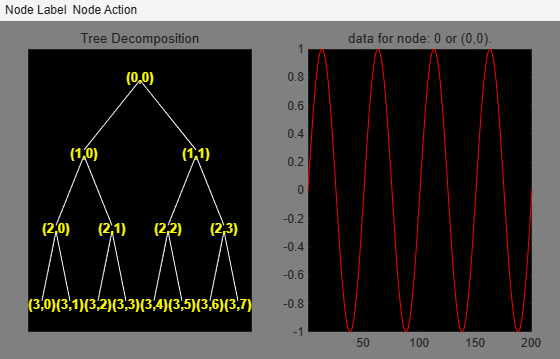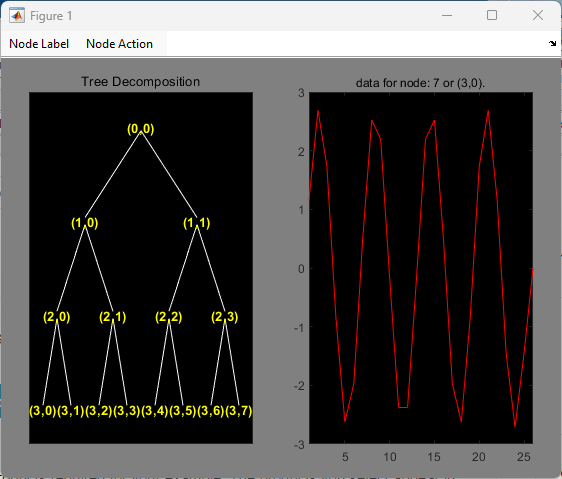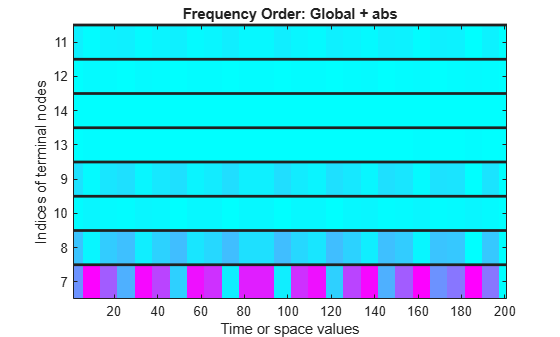# wpviewcf

Plot wavelet packets colored coefficients

## Syntax

``wpviewcf(T,cmode)``
``wpviewcf(T,cmode,nbcol)``

## Description

example

````wpviewcf(T,cmode)` plots the colored coefficients for the terminal nodes of the wavelet packet tree `T` using the color mode `cmode`.```
````wpviewcf(T,cmode,nbcol)` uses `nbcol` colors.```

## Examples

collapse all

Create a wavelet packet tree using the Haar wavelet.

```Fs = 200; x = 0:1/Fs:1; y = sin(8*pi*x); t = wpdec(y,3,"haar");```

Use `plot`, the plot tree GUI, to plot the tree.

`plot(t)`Click the node `(3,0)` to get this figure:Plot the colored wavelet packet coefficients.

`wpviewcf(t,1)`## Input Arguments

collapse all

Wavelet packet tree, specified as a `wptree` object.

Color mode to use to plot the coefficients, specified by one of the values listed here.

Color ModeDescription
`1`Frequency order – Global coloration – Absolute values
`2`Frequency order – By level – Absolute values
`3`Frequency order – Global coloration – Values
`4`Frequency order – By level coloration – Values
`5`Natural order – Global coloration – Absolute values
`6`Natural order – By level – Absolute values
`7`Natural order – Global coloration – Values
`8`Natural order – By level coloration – Values

Number of colors to use to plot the coefficients, specified as a positive integer.

## Version History

Introduced before R2006a